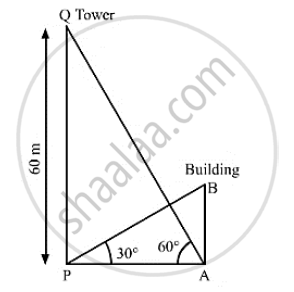# The Angle of Elevation on the Top of a Building from Foot of Tower is 30° . the Angle of Elevation of the Top of Tower When Seen from the Top of Second Water is 60° ., Find Height of the Building. - Mathematics

The angle of elevation on the top of a building from the foot of a tower is 30° . The angle of elevation of the top of the tower when seen from the top of the second water is 60° .If the tower is 60m high, find the height of the building.

#### SolutionLet AB be thee building and PQ be the tower.
We have,

PQ = 60m,∠APB = 30°, ∠PAQ = 60°
In  ΔAPQ,

 tan 60° = (PQ)/(AP)

⇒ sqrt(3) = 60/(AP)

⇒ AP = 60/sqrt(3)

⇒ AP = (60 sqrt(3))/3

⇒ AP = 20 sqrt(3) m

Now , in Δ ABP ,

  tan 30° = (AB)/(AP)

⇒1/ sqrt(3) = (AB)/(20 sqrt(3))

⇒ AB = (20 sqrt(3))/ sqrt(3)

∴ AB = 20 m

So, the height of the building is 20 m

Concept: Heights and Distances
Is there an error in this question or solution?
Chapter 14: Height and Distance - Exercises

#### APPEARS IN

RS Aggarwal Secondary School Class 10 Maths
Chapter 14 Height and Distance
Exercises | Q 16

Share